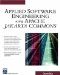# Step 3: Analyzing a Completed Action

Next you need to consider the total time required to implement and debug the action. The problem with tracking these times for an action is that a programmer or QA team member must track how long they work on this. This can seem to be like filling out a time card, and knowledge workers who are part of a team don't usually like time cards. Therefore, your team members may resist. A good solution to this problem is not to use a time card but to have bi-weekly update meetings. The team leader or auditor manages these meetings, and he goes through the action items to see what work needs to be completed. This approach puts an individual touch on things, and it does not require the programmer or QA team member to track his own time.

The total time taken to implement and debug are represented as attributes of the action XML tag: timeImpl and debugTime . Once you come up with these values, you compare them to the time to implement and risk in implementation. At this point, the risk is in the form of a numeric value that indicates how risky the action is. We'll convert it into a time using Equation A.4.

(A.4)` [time to implement] + (e([risk of time to implement]) / e([risk of time to implement] / [constant])) `The calculation uses the logarithmic function to calculate the risk. A logarithmic function reflects how we think. For example, if the risk indicates that the actual time to implement should be an extra couple of time units, then it is easy to judge. However, a risk of 10, which for this book is considered maximum, could add months or years to the project. This sudden jump from days to months or years is consistent with how humans think. Humans can assess low risk well, but not large risks. Large risks involve too much time and other variables . The e logarithmic function exponentially increases its result as the input value increases . The only problem with this way of calculating is that if the value of e is 10, a value of 22,000 results; this is way too high. The problem is that the exponential curve swings too high, too fast. The solution is to divide the e result with another e result that is modified by a constant as shown in Equation A.4. Note the variable in the entire equation is the true value of the constant variable. This is a real number between one and two and is found by tracking your own development process.We now use Equation A.5 to do a post-mortem analysis of the action.

(A.5)` [time calculated with risk] - ([time to implement] + [time to debug]) `The end result of Equation A.5 is a time difference between the actual time and the predicted one. Typically there will be a difference. The goal is to make the difference as small as possible. The way to do that is to create a feedback loop that fixes up your assessments for the future.

Mathematical statistics help us determine which actions to focus on. Using statistics, we can calculate a variance, and based on that variance we can pinpoint specific actions as problem actions. The age-old rule that 80 percent of the problems are caused by 20 percent of the actions is absolutely correct. In statistics, the rule roughly approximates to a standard deviation. A standard deviation assumes that the data falls into a bell curve. The bell curve says that 66.7 percent of the data is within one standard deviation. Ninety-five percent of the data is contained in two standard deviations, and 99.7 percent of the data is contained in three standard deviations.

To find the problematic actions, find all actions where the calculated time to implement and actual time to implement is greater than one standard deviation away from the calculated average. Note that this proposed calculation is not saying, "find all actions that were not implemented in a reasonable amount of time, but find the actions that gave the most problems." Finding those problematic actions allows a developer to find out what went wrong. To calculate the standard deviation, we use Equation A.6, which calculates the median value of the differences.

(A.6)` 1 / [n] *([difference 1] + ... + [difference n]) `Once you have determined the median value, you can use Equation A.7 to calculate the standard deviation.

(A.7)` SQRT(1 / (n  1) * (POW([difference 1]  [median], 2) + .. +  POW([difference n]  [median], 2)) `Applied Software Engineering Using Apache Jakarta Commons (Charles River Media Computer Engineering)
ISBN: 1584502460
EAN: 2147483647
Year: 2002
Pages: 109